• 25
•
• 1
•
•
•
•
•
26
Shares

# Work-Energy Theorem

Today, we are going to derive the work-energy theorem and its derivation is universally applicable for all constant and variable forces and for translation and rotational work.## Work-Energy Theorem

### For translation work

Work Energy theorem in some books also referred to as work and energy principle is basically a theorem stating that the sum of the translational work done by each force including internal and external forces on a system Is equals to the change in translation kinetic energy of the system. Don’t worry stay tuned we’d proved it below.

### For Rotational work

Similarly, the sum of all rotational work done by each force internal or external on a system equals the change in the rotational kinetic energy of the system. It’s also proved below.

## Derivation of Work energy theorem

### For the system in translation motion

Any system in translational motion possess energy as the system in motion are capable of doing work, this energy due to translational motion is translational kinetic energy also known as kinetic energy, and defined as-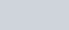K, where means translational kinetic energy. Now, differentiating both sides w.r.t time t.As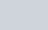Here v is speed, not velocity. And the change in speed is only due to the tangential component of acceleration as acceleration perpendicular to speed (direction of motion) won’t change the speed it will affect the direction only.

(1)Also

(2)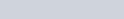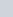is tangential component of force , force which is in direction of tangential acceleration .
From eq (1) and eq (2), we get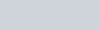(3)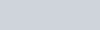Also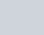(4)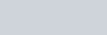(5)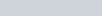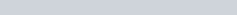(6)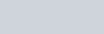Here,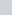is the net force on the system under study and is the vector sum of all forces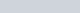, etc acting on the system, whether internal or external.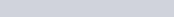Now equation (6) becomes

(7)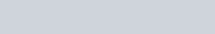(8)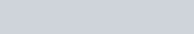The R.H.S of equation (8) is the sum of translational work done by each force acting on the system, and L.H.S is changed in the kinetic energy of the system. and this equation is a work-energy theorem which we can state as :

Statement – The sum of the work done by each force acting on a system whether internal or external is equal to change in the kinetic energy of the system this is work-energy-theorem for the system in translational motion.

### For System in rotational motion

Any system in rotational motion is capable of doing work hence said to be possessing energy and this energy is known as rotational kinetic energy and is defined as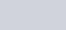Hereand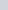are angular velocity and moment of Inertia respectively.
Differentiate both sides w.r.t time

(9)Since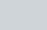means angular acceleration. We know that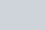and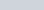(tau) which means net torque, therefore Equation (9) becomes as

(10)(11)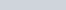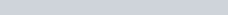(12)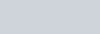(13)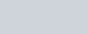here is the net torque and is obtained by vector sum of torque due to every force whether internal or external.

(14)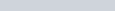Hence equation (14) becomes

(15)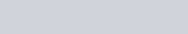The R.H.S of equation (15) is the sum of rotational work done by each force acting on the system, and L.H.S is changed in rotational kinetic energy of the system. and this equation is work-energy theorem governing the rotational motion of the system which we can state as :

Statement – The sum of the rotational work done by each force acting on a system whether internal or external is equals to change in the rotational kinetic energy of system this is work-energy-theorem for system in rotational motion.

Stay tuned with Laws Of Nature for more useful and interesting content.